## Introduction

• There are four different types of transformations.
1. Dilations
2. Reflections
3. Rotations
4. Translations
• To transform a figure (shape) means changing the size, location, and direction it faces.
• The figure before the transformation occurs is called the pre-image.
• The figure after the transformation is called the image.
• If the pre-image is labeled as A, then the image would then be labeled as A' (pronounced as "A prime").

## Congruency

• Two images are congruent if they are of the same shape and size.
• The image after translation is congruent to the pre-image.
• Translations are rigid motions. Rigid motions preserve distance (side lengths) and angle measures.
• The symbol for congruency is ≅.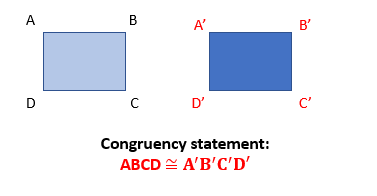## Translation

• A translation moves (slides) or displaces every point of the figure by the same distance and in the same direction
• Translation in coordinate plane:
• In translation, the figure slides but never turns or rotates.
• In translation, pre-image and image are congruent.
• The figure can slide in any direction on the coordinate plane.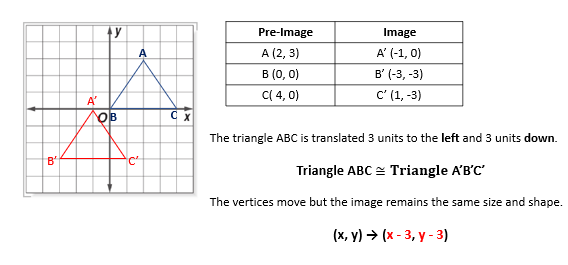## Solved Examples

Question 1: $△{A}^{\text{'}}{B}^{\text{'}}{C}^{\text{'}}$ is the translated image of $△ABC$. What is the translation rule that models the given transformation?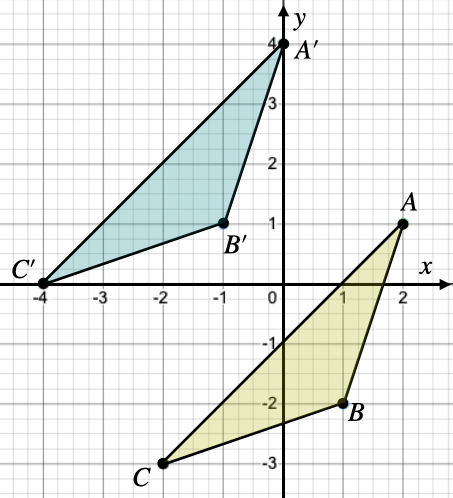Solution: From the graph, it is evident that each point of the pre-image is shifted 2 units to the left and 3 units upwards. Hence, the translation rule is

Question 2: The coordinates of a quadrilateral are shown in the table below. If the image has ${A}^{\text{'}}$ at a point $\left(-1,7\right)$, what are the coordinates of ${B}^{\text{'}}$,${C}^{\text{'}}$ and ${D}^{\text{'}}$?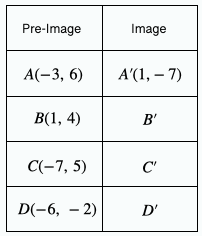Solution: To find the coordinates for these points, first identify the translation that moves the point $A$ to ${A}^{\text{'}}$. The x-coordinate moves from -3 to 1, so the horizontal movement is $\left(x+4\right)$. The y-coordinate moves from 6 to -7, so the vertical movement is $\left(y-13\right)$. Therefore, we want to translate each coordinate as .

The coordinates of ${B}^{\text{'}}$ are $x=\left(1+4\right)=5$, and $y=\left(4-13\right)=-9$. Point ${B}^{\text{'}}$ is at .

The coordinates of ${C}^{\text{'}}$ are $x=\left(-7+4\right)=-3$, and $y=\left(-5-13\right)=-18$. Point ${B}^{\text{'}}$ is at .

The coordinates of ${D}^{\text{'}}$ are $x=\left(-6+4\right)=-2$, and $y=\left(-2-13\right)=-15$. Point ${B}^{\text{'}}$ is at .

## Cheat Sheet

• The algebraic representation of a translation depends on the directions of the displacement.
• When the $x-$values are changed, the figure moves horizontally (left or right).
• When the $y-$values are changed, the figure moves vertically (up or down).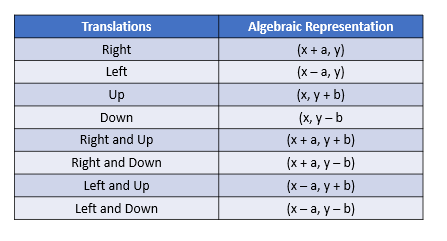## Blunder Areas

• Differentiate between pre-image (A) and image (A').
• Think of the movements in terms of positive and negative.
• Movements to the right and up are positive.
• Movements to the left and down are negative.
• Horizontal (left and right) movements change the $x-$values.
• Vertical (up and down) movements change the $y-$values.